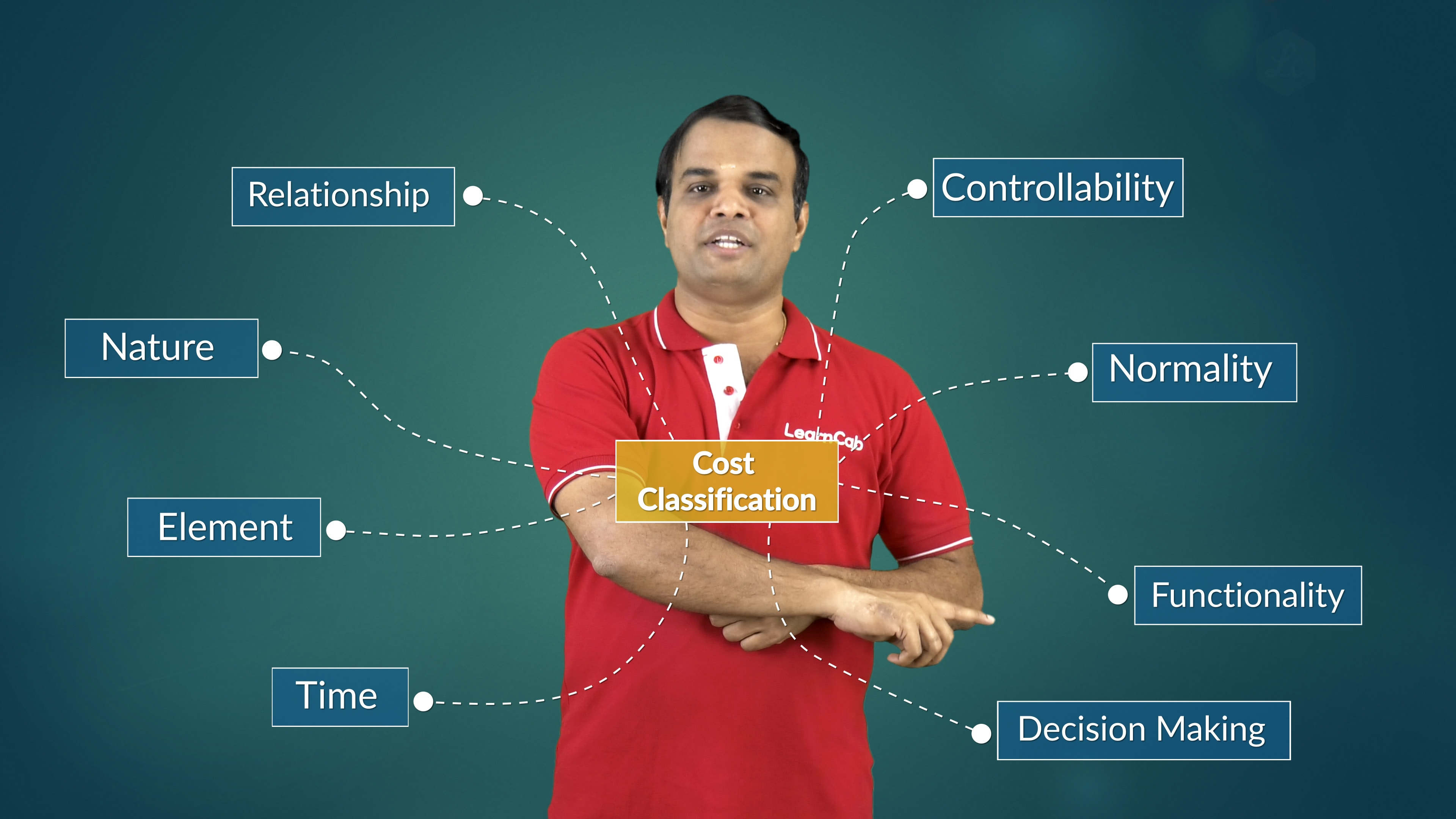# Why is Costing so Difficult in CA Intermediate?So, it is quite clear that many students face extreme difficulty in the costing paper in CA Intermediate.In fact, students face difficulty in understanding the questions in the paper itself! They have a problem with the problems!

But really, this should not be the case.

If you have a thorough understanding of the concepts, you should face no difficulty. Costing = common sense! Let that be your mantra.

The problem (if I can use this word again!) is that the concepts in costing are not all that difficult. So, you think that the paper is going to be easy. And this means you don’t end up revising the concepts completely.

And this leads to difficulty in understanding the questions in the paper!

Let us go over, very briefly, the concepts involved in costing.

### What is costing?

Costing is nothing but the process of ascertaining or determining the expenditure incurred for producing a product or rendering of a service. This is from the manufacturer’s point of view and not from the end user.

This process helps you to understand in depth where your major costs are incurred. And how to manage such costs.

Before we go further into details of how to classify costs, let us look at the reason why costing is needed. After all, you should know why one should do a thing before doing it!

## Why is costing necessary?

Costing is needed for many reasons. Some of the following are:

• Cost ascertainment: Determining the costs is necessary so you understand where your major costs are incurred!
• Determination of selling price: Understanding how much the cost of producing a product or rendering of the service helps you to determine a suitable selling price
• Ascertaining profit: It helps you to identify how much profit you might make depending on your selling price (which in turn is based on your cost of manufacture or rendering of service!)
• Cost control: You will be able to identify which aspects of your manufacture, etc., cost more. This will lead to effective cost control
• Decision-making: The whole process helps you to make decisions on when and where to save on costs

Now, let’s look at how to classify the costing process.

## Cost classification

Cost can be classified into the following:

• Time based: This can be further classified into historical cost, current cost and pre-determined cost
• Element: This involves the material labour and other expenditure
• Nature (behaviour, etc.): This is to do with fixed cost, variable cost and semi-variable cost
• Relationship: Both direct and indirect
• Controllability: Involves both controllable and uncontrollable costs
• Normality: both normal and abnormal
• Functionality: Involves everything from production, administration, selling and distribution, research and development to pre-production and conversion costs
• Decision-making: Both relevant and irrelevant situations

The chart below sums all this up nicely:A few final remarks

This concludes our very brief survey of costing. The takeaway is that if you know the concepts involved thoroughly, you should face no difficulty in solving the paper.

After all, the mathematics involved is very simple too! Just simple addition, subtraction, multiplication and division. Okay, occasionally square root.

The trick is to understand when you should add, subtract, multiply or divide.

If you can figure out this trick, costing = common sense.

Want more proof of why costing = common sense? Look atwho explains why costing = common sense, and why students face difficulty.